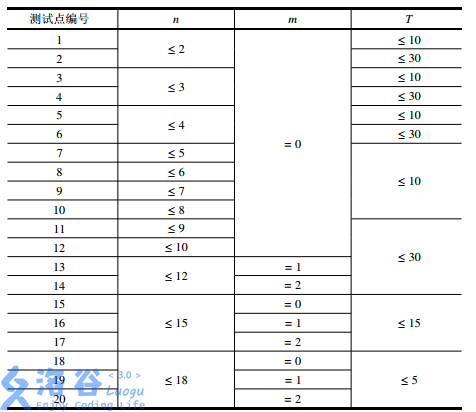# P2831 愤怒的小鸟

• 5.1K通过
• 20.1K提交
• 题目提供者 CCF_NOI
• 评测方式 云端评测
• 标签 搜索 NOIp提高组 2016 高性能
• 难度 提高+/省选-
• 时空限制 2000ms / 512MB
• 提示：收藏到任务计划后，可在首页查看。

## 题目描述

Kiana 最近沉迷于一款神奇的游戏无法自拔。

简单来说，这款游戏是在一个平面上进行的。

有一架弹弓位于 $(0,0)$ 处，每次 Kiana 可以用它向第一象限发射一只红色的小鸟，小鸟们的飞行轨迹均为形如 $y=ax^2+bx$ 的曲线，其中 $a,b$ 是Kiana 指定的参数，且必须满足 $a < 0$，$a,b$ 都是实数。

当小鸟落回地面（即 $x$ 轴）时，它就会瞬间消失。

在游戏的某个关卡里，平面的第一象限中有 $n$ 只绿色的小猪，其中第 $i$ 只小猪所在的坐标为 $\left(x_i,y_i \right)$。

如果某只小鸟的飞行轨迹经过了 $\left( x_i, y_i \right)$，那么第 $i$ 只小猪就会被消灭掉，同时小鸟将会沿着原先的轨迹继续飞行；

如果一只小鸟的飞行轨迹没有经过 $\left( x_i, y_i \right)$，那么这只小鸟飞行的全过程就不会对第 $i$ 只小猪产生任何影响。

例如，若两只小猪分别位于 $(1,3)$ 和 $(3,3)$，Kiana 可以选择发射一只飞行轨迹为 $y=-x^2+4x$ 的小鸟，这样两只小猪就会被这只小鸟一起消灭。

而这个游戏的目的，就是通过发射小鸟消灭所有的小猪。

这款神奇游戏的每个关卡对 Kiana来说都很难，所以Kiana还输入了一些神秘的指令，使得自己能更轻松地完成这个游戏。这些指令将在【输入格式】中详述。

假设这款游戏一共有 $T$ 个关卡，现在 Kiana想知道，对于每一个关卡，至少需要发射多少只小鸟才能消灭所有的小猪。由于她不会算，所以希望由你告诉她。

## 输入输出格式

输入格式：

第一行包含一个正整数 $T$，表示游戏的关卡总数。

下面依次输入这 $T$ 个关卡的信息。每个关卡第一行包含两个非负整数 $n,m$，分别表示该关卡中的小猪数量和 Kiana 输入的神秘指令类型。接下来的 $n$ 行中，第 $i$ 行包含两个正实数 $x_i,y_i$，表示第 $i$ 只小猪坐标为 $(x_i,y_i)$。数据保证同一个关卡中不存在两只坐标完全相同的小猪。

如果 $m=0$，表示Kiana输入了一个没有任何作用的指令。

如果 $m=1$，则这个关卡将会满足：至多用 $\lceil n/3 + 1 \rceil$ 只小鸟即可消灭所有小猪。

如果 $m=2$，则这个关卡将会满足：一定存在一种最优解，其中有一只小鸟消灭了至少 $\lfloor n/3 \rfloor$ 只小猪。

保证 $1\leq n \leq 18$，$0\leq m \leq 2$，$0 < x_i,y_i < 10$，输入中的实数均保留到小数点后两位。

上文中，符号 $\lceil c \rceil$ 和 $\lfloor c \rfloor$ 分别表示对 $c$ 向上取整和向下取整，例如：$\lceil 2.1 \rceil = \lceil 2.9 \rceil = \lceil 3.0 \rceil = \lfloor 3.0 \rfloor = \lfloor 3.1 \rfloor = \lfloor 3.9 \rfloor = 3$。

输出格式：

对每个关卡依次输出一行答案。

输出的每一行包含一个正整数，表示相应的关卡中，消灭所有小猪最少需要的小鸟数量。

## 输入输出样例

输入样例#1： 复制
2
2 0
1.00 3.00
3.00 3.00
5 2
1.00 5.00
2.00 8.00
3.00 9.00
4.00 8.00
5.00 5.00
输出样例#1： 复制
1
1
输入样例#2： 复制
3
2 0
1.41 2.00
1.73 3.00
3 0
1.11 1.41
2.34 1.79
2.98 1.49
5 0
2.72 2.72
2.72 3.14
3.14 2.72
3.14 3.14
5.00 5.00
输出样例#2： 复制
2
2
3

输入样例#3： 复制
1
10 0
7.16 6.28
2.02 0.38
8.33 7.78
7.68 2.09
7.46 7.86
5.77 7.44
8.24 6.72
4.42 5.11
5.42 7.79
8.15 4.99
输出样例#3： 复制
6


## 说明

【样例解释1】

这组数据中一共有两个关卡。

第一个关卡与【问题描述】中的情形相同，$2$只小猪分别位于$(1.00,3.00)$和 $(3.00,3.00)$，只需发射一只飞行轨迹为$y = -x^2 + 4x$的小鸟即可消灭它们。

第二个关卡中有$5$只小猪，但经过观察我们可以发现它们的坐标都在抛物线 $y = -x^2 + 6x$上，故Kiana只需要发射一只小鸟即可消灭所有小猪。

【数据范围】提示
标程仅供做题后或实在无思路时参考。
请自觉、自律地使用该功能并请对自己的学习负责。
如果发现恶意抄袭标程，将按照I类违反进行处理。